# NCERT Solutions For Class 10 Maths Chapter 11 Exercise 11.2

Go back to  'Constructions'

## Chapter 11 Ex.11.2 Question 1

Draw a circle of radius $$6 \,\rm{cm}$$. From a point $$10\,\rm{cm}$$ away from its centre, construct the pair of tangents to the circle and measure their lengths.

#### Steps: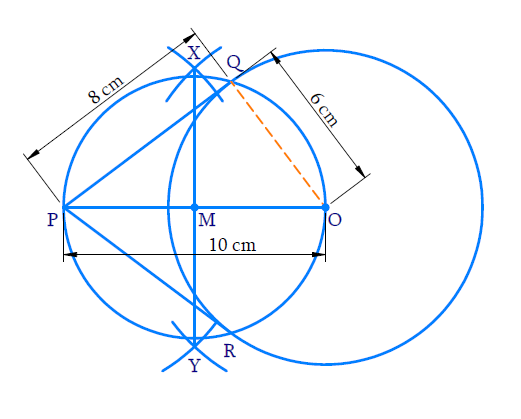Steps of construction:

(i) Take a point $$O$$ as centre and $$6 \,\rm{cm}$$ radius. Draw a circle.

(ii) Take a point $$P$$ such that $$OP =$$ $$10\,\rm{cm}$$.

(iii) With $$O$$ and $$P$$ as centres and radius more than half of $$OP$$ draw arcs above and below $$OP$$ to intersect at $$X$$ and $$Y.$$

(iv) Join $$XY$$ to intersect $$OP$$ at $$M$$.

(v) With $$M$$ as centre and $$OM$$ as radius draw a circle to intersect the given circle at $$Q$$ and $$R$$.

(vi) Join $$PQ$$ and $$PR$$.

$$PQ$$ and $$PR$$ are the required tangents where\begin{align}\rm{PQ}=\rm{PR}=8 \rm{cm.}\end{align}

Proof:

$$\angle {{PQO}} = {90^ \circ } \Rightarrow {\rm{PQ}} \bot {\rm{OQ}}$$ (Angle in a semicircle)

$$OQ$$ being the radius of the given circle, $$PQ$$ in the tangent at $$Q.$$

In right $${{\Delta PQO,}}$$

$${{OP = 10 \,\rm{cm,} OQ = 6}}\,{\rm{cm}}$$ (radius)

\begin{align} {{P}}{{{Q}}^{{2}}} &={{O}}{{{P}}^{{2}}}{{ - O}}{{{Q}}^{{2}}}\\ &= {{ (10}}{{{)}}^{{2}}}{{ - (6}}{{{)}}^{{2}}}\\ &= { {100 - 36}}\\ &= {{ 64}} \end{align}

\begin{align} {{PQ }}&=\sqrt {{{64}}} \\ &= {{8}}\,{\rm{cm}} \end{align}

Similarly, $$PR =$$ $$8 \;\rm{cm}$$.

## Chapter 11 Ex.11.2 Question 2

Construct a tangent to a circle of radius $$4 \,\rm{cm}$$ from a point on the concentric circle of radius $$6 \,\rm{cm}$$ and measure its length. Also verify the measurement by actual calculation.

#### Steps: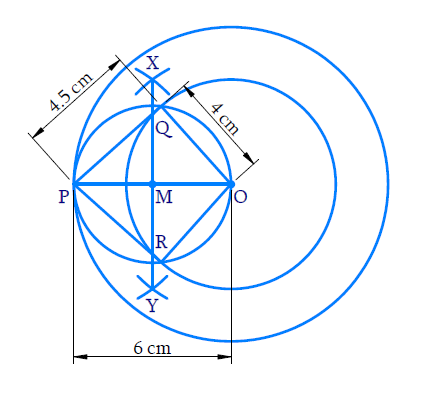Steps of construction:

(i) Take $$‘O’$$ as centre and radius $$4 \,\rm{cm}$$ and $$6\,\rm{cm}$$ draw two circles.

(ii) Take a point $$‘P’$$ on the bigger circle and join $$OP$$.

(iii) With $$‘O’$$ and $$‘P’$$ as centre and radius more than half of $$OP$$ draw arcs above and below $$OP$$ to intersect at $$X$$ and $$Y$$.

(iv) Join $$XY$$ to intersect $$OP$$ at $$M$$.

(v) With $$M$$ as centre and $$OM$$ as radius draw a circle to cut the smaller circle at $$Q$$ and $$R.$$

(vi) Join $$PQ$$ and $$PR$$.

$$PQ$$ and $$PR$$ are the required tangent where \begin{align}\rm{PQ}=4.5\end{align} (aprox)

Proof:

$$\angle {\rm{PQO}} = {90^ \circ }$$ (Angle in a semi - circle)

$$\therefore$$\begin{align} P Q \perp O Q\end{align}

$$OQ$$ being the radius of the smaller circle, $$PQ$$ is the tangent at $$Q$$.

In right $${\rm{\Delta PQO,}}$$

$$OP = 6\,\rm{cm}$$ (radius of the bigger circle)

$$OQ = 4 \,\rm{cm}$$ (radius of the smaller circle)

\begin{align} {PQ}^{2} &=({OP})^{2}-(\mathrm{OQ})^{2} \\ &=(6)^{2}-(4)^{2} \\ &=36-16 \\ &=20 \end{align}

\begin{align} {{PQ }}&=\sqrt {{\rm{20}}} \\ &={{4.5}}\,\,\,{\rm{(approx)}} \end{align}
Similarly, $${\rm{PR = 4}}{\rm{.5 }}\left( {{\rm{approx}}{\rm{.}}} \right)$$

## Chapter 11 Ex.11.2 Question 3

Draw a circle of radius $$3 \,\rm{cm}$$. Take two points $$P$$ and $$Q$$ on one of its extended diameter each at a distance of $$7 \,\rm{cm}$$ from its center. Draw tangents to the circle from these two points $$P$$ and $$Q$$.

#### Steps: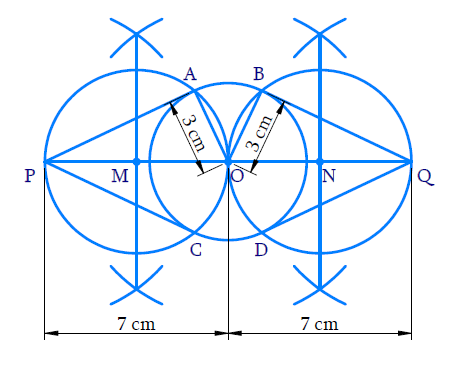Steps of construction:

(i) Draw a circle with $$O$$ as centre and radius is $$3 \,\rm{cm}$$.

(ii) Draw a diameter of it extend both the sides and take points $$P$$, $$Q$$ on the diameter such that \begin{align}\rm{OP}=\rm{OQ}=7 \rm{cm}\end{align}

(iii) Draw the perpendicular bisectors of $$OP$$ and $$OQ$$ to intersect $$OP$$ and $$OQ$$ at $$M$$ and $$N$$ respectively.

(iv) With $$M$$ as centre and $$OM$$ as radius draw a circle to cut the given circle at $$A$$ and $$C$$. With $$N$$ as centre and $$ON$$ as radius draw a circle to cut the given circle at $$B$$ and $$D$$.

(v) Join $$PA$$, $$PC$$ $$QB$$, $$QD$$

$$PA$$,$$PC$$ and $$QB$$,$$QD$$ are the required tangents from $$P$$ and $$Q$$ respectively.

Proof:

$$\angle {{PAO}} =\angle {{QBO}}=90^\circ$$ (Angle in a semi - circle)

$$\therefore {PA} \perp {AO},\; {QB} \perp {BO}$$

Since $$OA$$ and $$OB$$ are the radii of the given circle, $$PA$$ and QB are its tangents at $$A$$ and $$B$$ respectively.

In right angle triangle $$PAO$$ and $$QBO$$

$$OP = OQ = 7 \,\rm{cm }$$ (By construction)

$$OA = OB = 3\,\rm{cm}$$ (radius of the given circle)

\begin{align} {{P}}{{{A}}^{{2}}}&={{ (OP}}{{{)}}^{{2}}}{{ -(OA}}{{{)}}^{{2}}}\,\,\,\,\,\,\,\\ &= {{ (7}}{{{)}}^{{2}}}{{ - (3}}{{{)}}^{{2}}}\\ &= {{ 49 - 9}}\\ &={{ 40}}\\ {{PA }}&=\sqrt {{{40}}} \\ &={{ 6}}{{.3}}\,\,{\rm{(approx)}} \end{align}

And

\begin{align} {{Q}}{{{B}}^{{2}}}&={{(OQ}}{{{)}}^{{2}}}{{ - (OB}}{{{)}}^{{2}}}\,\,\,\,\,\,\,\\ &={{(7}}{{{)}}^{{2}}}{{ - (3}}{{{)}}^{{2}}}\\ &={{ 49 - 9}}\\ &={{ 40}}\\ {{QB }}&=\sqrt {{{40}}} \\ &={{ 6}}{{.3}}\,\,{\rm{(approx)}} \end{align}

## Chapter 11 Ex.11.2 Question 4

Draw a pair of tangents to a circle of radius $$5 \,\rm{cm}$$ which are inclined to each other at an angle of $$60^\circ$$.

#### Steps: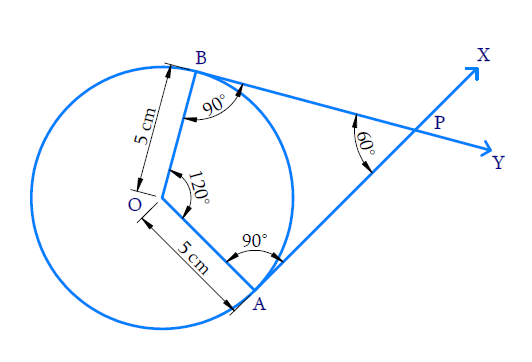Steps of construction:

(i)    With $$O$$ as centre and $$5 \,\rm{cm}$$ as radius draw a circle.

(ii)    Take a point $$A$$ on the circumference of the circle and join $$OA$$.

(iii)   Draw $$AX$$ perpendicular to $$OA$$.

(iv)   Construct \begin{align}\angle \rm{AOB}=120^{\circ} \end{align} where $$B$$ lies on the circumference.

(v)    Draw $$BY$$ perpendicular to $$OB$$.

(vi)   Both $$AX$$ and $$BY$$ intersect at $$P$$.

(vii)  $$PA$$ and $$PB$$ are the required tangents inclined at \begin{align}60^{\circ}\end{align} .

Proof:

$$\angle {{OAP}} = \angle {{OBP}} = 90^\circ$$ (By construction)

$$\angle {{AOB}} = 120^\circ$$ (By construction)

In quadrilateral $$OAPB$$,

\begin{align}\angle APB&\!=\!{{360}^{{}^\circ }}\!-\!\!\left[ \begin{array}& \angle OAP\!+\!\angle OBP+ \\ \angle AOB \\ \end{array}\!\right] \\ & \!=\!{{360}^{^\circ }}\!-\!\![{{90}^{^\circ }}+{{90}^{^\circ }}+{{120}^{{}^\circ }}] \\ &\!=\!{{360}^{^\circ }}\!-\!\!{{300}^{^\circ }} \\ & \!=\!{{60}^{^\circ }} \\ \end{align}

Hence $$PA$$ and $$PB$$ are the required tangents inclined at \begin{align}60^{\circ}\end{align}.

## Chapter 11 Ex.11.2 Question 5

Draw a line segment $$AB$$ of length $$8 \,\rm{cm}$$. Taking $$A$$ as centre, draw a circle of radius $$4 \,\rm{cm}$$ and taking $$B$$ as center, draw another circle of radius $$3 \,\rm{cm}$$. Construct tangent to each circle from the center of the other circle.

#### Steps: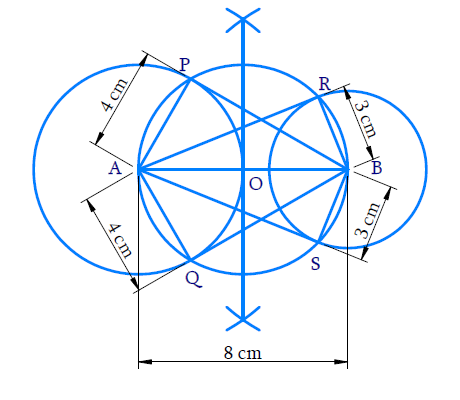Steps of construction:

(i) Draw \begin{align}\rm{AB}=8\, \rm{cm}\end{align}.With $$A$$ and $$B$$ as centers $$4 \,\rm{cm}$$ and $$3 \,\rm{cm}$$ as radius respectively draw two circles.

(ii)  Draw the perpendicular bisector of $$AB$$, intersecting $$AB$$ at $$O$$.

(iii) With $$O$$ as center and $$OA$$ as radius draw a circle which intersects the two circles at $$P$$, $$Q$$, $$R$$ and $$S$$.

(iv) Join $$BP$$, $$BQ$$, $$AR$$ and $$AS$$.

(v) $$BP$$ and $$BQ$$ are the tangents from $$B$$ to the circle with center $$A$$$$AR$$ and $$AS$$ are the tangents from $$A$$ to the circle with center $$B$$.

Proof:

$$\angle {APB}=\angle {AQB}=90^{\circ}$$ (Angle in a semi-circle)

$$\therefore \rm{AP} \perp \rm{PB}$$ and $${AQ} \perp {QB}$$

Therefore, $$BP$$ and $$BQ$$ are the tangents to the circle with center $$A$$.

Similarly, $$AR$$ and $$AS$$ are the tangents to the circle with center $$B$$.

## Chapter 11 Ex.11.2 Question 6

Let $$ABC$$ be a right triangle in which $$AB = 6 \,\rm{cm},$$ $$BC = 8 \,\rm{cm}$$ and \begin{align}\angle \rm{B}=90^{\circ}.\end{align}  $$BD$$ is the perpendicular to $$AC$$. The circle through $$B$$, $$C$$ and $$D$$ is drawn. Construct the tangents from $$A$$ to this circle.

#### Steps: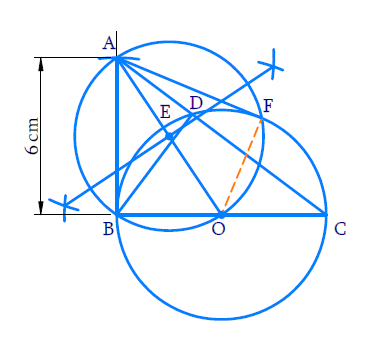Steps of construction:

(i) Draw \begin{align}\rm{BC}=8 \rm{cm}\end{align}. Draw the perpendicular at $$B$$ and cut \begin{align}\rm{BA}=6 \rm{cm}\end{align}on it. Join $$AC$$ right \begin{align}\triangle {ABC}\end{align}  is obtained.

(ii) Draw $$BD$$ perpendicular to $$AC$$.

(iii) Since \begin{align}\angle \rm{BDC}=90^{\circ}\end{align} and the circle has to pass through $$B, C$$ and $$D.$$ $$BC$$ must be a diameter of this circle. So, take $$O$$ as the midpoint of $$BC$$ and with $$O$$ as centre and $$OB$$ as radius draw a circle which will pass through $$B$$, $$C$$ and $$D$$.

(iv) To draw tangents from $$A$$ to the circle with center $$O$$.

(a) Join $$OA$$, and draw its perpendicular bisectors to intersect $$OA$$ at $$E$$.

(b) With $$E$$ as center and $$EA$$ as radius draw a circle which intersects the  previous circle at $$B$$ and $$F$$.

(c) Join $$AF$$.

$$AB$$ and $$AF$$ are the required tangents.

Proof:

$$\angle {{ABO}} = \angle {{AFO}} = 90^\circ$$  ( Angle in a semi $$-$$ circle)

$$\therefore$$ $${AB} \bot {OB}$$ and $${AF} \bot {OF}$$

Hence $$AB$$ and $$AF$$ are the tangents from $$A$$ to the circle with centre $$O$$.

## Chapter 11 Ex.11.2 Question 7

Draw a circle with the help of a bangle. Take a point outside the circle. Construct the pair of tangents from this point to the circle.

### Solution

Steps: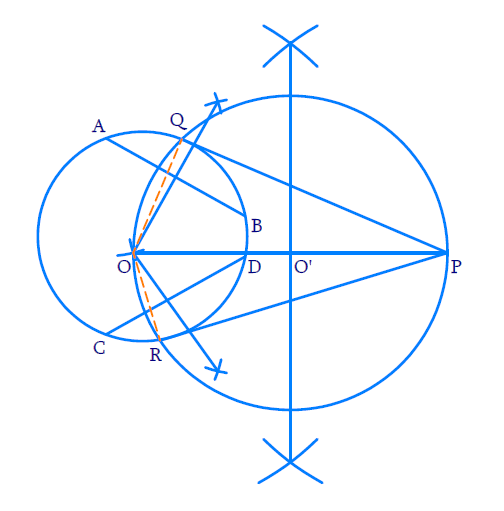Steps of construction:

(i)  Draw any circle using a bangle.

To find its centre

(a) Draw any two chords of the circle say $$AB$$ and $$CD$$.

(b) Draw the perpendicular bisectors of $$AB$$ and $$CD$$ to intersect at $$O$$.

Now, $$‘O’$$ is the centre of this circle (since the perpendiculars drawn from the centre of a circle to any chord bisect the chord and vice versa).

To draw the tangents from a point $$‘P’$$ outside the circle.

(ii) Take any point $$P$$ outside the circle and draw the perpendicular bisector of $$OP$$ which meets at $$OP$$ at $$O’$$.

(iii) With $$O’$$ as center and $$OO’$$ as radius draw a circle which cuts the given circle at $$Q$$ and $$R$$.

(iv) Join $$PQ$$ and $$PR$$.

$$PQ$$ and $$PR$$ are the required tangents.

Proof:

$$\angle {\rm{OQP}} = \angle {\rm{ORP}} = 90^\circ$$ (Angle in a semi $$-$$ circle)

$$\therefore \,{OQ} \,\bot\, {QP}$$ and $${OR}\, \bot\, {RP}$$

Hence, $$PQ$$ and $$PR$$ are the tangents to the given circle.